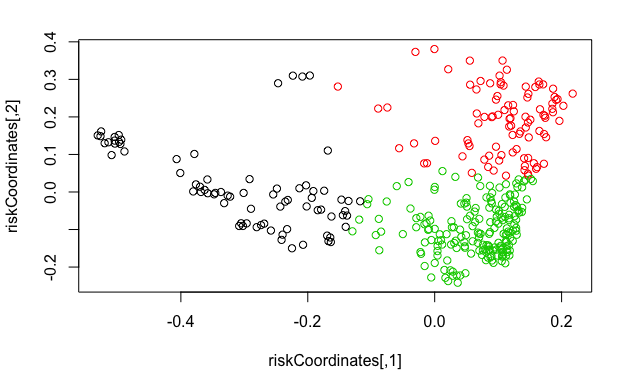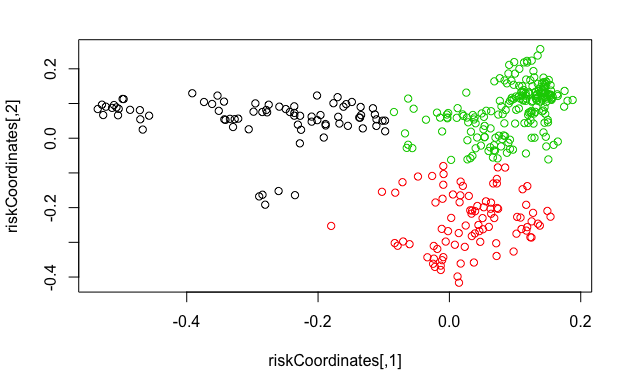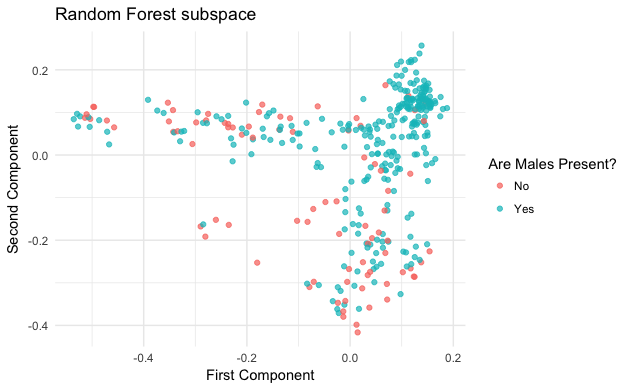``````library(magrittr) # for pipe programming
library(randomForest) # implementation of the RF algorithm``````
``````randomForest 4.6-12
Type rfNews() to see new features/changes/bug fixes.``````
``library(ggplot2) # library for graphics``
``````
Attaching package: ‘ggplot2’

The following object is masked from ‘package:randomForest’:

margin``````
``Cropfeedingbouts <- read_excel("~/ACADEMY/Papers/Bipedalism, Chimps, Lovejoy/Cropfeedingbouts.xlsx")``

## 1. Data recoding and reorganization:

``````cp0 <- data.frame(Cropfeedingbouts[,-1])
cp1 <- cp0[,c(3:5)] # getting numeric variables
cp2 <- cp0[,-c(3:5,15)] # remove numeric variables, and "first to get there ID"" as well
cp2\$Guard[cp2\$Guard == 3] <- -1 # might be useful later for the risk of Guard variable to be ordered.
INDIVIDUALS <- data.matrix(cp2[,c(16:27)]) # matrix of present individuals
cp2\$Distance[is.na(cp2\$Distance)] <- 0 # Removing NAs
# checking for errors in number of males and party size:
table(rowSums(INDIVIDUALS[,1:3]) == cp2\$NoMales) # 4 errors``````
``````
FALSE  TRUE
4   361 ``````
``table(rowSums(INDIVIDUALS) == cp2\$Partysize)    # 6 errors``
``````
FALSE  TRUE
6   359 ``````
``````# Since there are errors we need to rebuild the variables. I will use the matrix of present individuals.
cp2\$NoMales <- rowSums(INDIVIDUALS[,1:3])
cp2\$Partysize <- rowSums(INDIVIDUALS)
# Transform all variables from cp2 (which as no true numericals already) into factors!
cp2[] <- lapply(cp2, factor)
# rebuilt dataset without individual entrances
cropData <- cbind(cp1, cp2[,c(1:15)])
# ALL ERRORS CORRECTED :-)
# Now, giving the correct coding for non-numeric variables, just to make graphics prettier and easier to understand:
levels(cropData\$Month) <- c(
'Jan', 'Feb', 'Mar', 'Apr', 'May', 'Jun',
'Jul', 'Aug', 'Sep', 'Oct', 'Nov', 'Dec')
levels(cropData\$HighLow) <- c('Low', 'High')
levels(cropData\$FdName) <- c(
'papaya', 'banana', 'orange', 'mandarin',
'pineapple', 'cassava', 'maize', 'cacao',
'oilpalm', 'raffia', 'okra', 'rice', 'mango',
'mixedcarb')
levels(cropData\$FdPart) <- c(
'fruit', 'leaf', 'pith', 'root', 'nut', 'gum',
'fruitleaf', 'fruitpith', 'fruitroot', 'leafpith',
'fruitnut', 'leafroot', 'pithgum', 'nutflower',
'fruitleafroot')
levels(cropData\$Guard) <- c(
'Lab simulation', 'Abandoned',
'Low human guard', 'High human guard')
levels(cropData\$People) <- c('Absent', 'Present')
levels(cropData\$ForestVillage) <- c('Forest', 'Village')
levels(cropData\$firstMale) <- c('No', 'Yes')
levels(cropData\$GpComp) <- c(
'male only', 'female only', 'female & immature',
'male & immature', 'mixed', 'male & female',
'immature')
levels(cropData\$Malspres) <- c('No', 'Yes')``````

## 2. Pre-processing

#### Creating “Risk Vectors”

``str(cropData)``
``````'data.frame':   365 obs. of  18 variables:
\$ StartTime    : num  710 710 713 740 741 745 800 808 809 840 ...
\$ EndTime      : num  807 NA 719 746 743 830 807 NA 838 841 ...
\$ Duration     : num  57 NA 6 6 2 45 7 NA 29 1 ...
\$ Month        : Factor w/ 12 levels "Jan","Feb","Mar",..: 1 1 1 1 1 1 1 1 1 1 ...
\$ HighLow      : Factor w/ 2 levels "Low","High": 2 2 2 2 2 2 2 2 2 2 ...
\$ Partysize    : Factor w/ 11 levels "1","2","3","4",..: 5 4 3 6 6 7 2 2 6 2 ...
\$ MaxParty     : Factor w/ 3 levels "12","13","14": 1 1 1 1 1 1 1 1 1 1 ...
\$ FdName       : Factor w/ 18 levels "papaya","banana",..: 9 12 9 12 12 9 9 18 9 12 ...
\$ FdPart       : Factor w/ 15 levels "fruit","leaf",..: 11 3 1 3 3 11 1 12 11 3 ...
\$ A.T          : Factor w/ 2 levels "A","T": 2 2 2 2 2 2 2 2 2 1 ...
\$ Guard        : Factor w/ 4 levels "Lab simulation",..: 1 2 1 2 4 1 1 4 1 2 ...
\$ People       : Factor w/ 2 levels "Absent","Present": 1 NA 1 1 1 1 1 NA 1 1 ...
\$ ForestVillage: Factor w/ 2 levels "Forest","Village": 1 2 1 2 1 1 1 1 1 1 ...
\$ Distance     : Factor w/ 15 levels "0","1","3","4",..: 1 5 1 5 1 1 1 1 1 1 ...
\$ firstMale    : Factor w/ 2 levels "No","Yes": 1 NA NA NA NA 2 1 2 2 1 ...
\$ GpComp       : Factor w/ 7 levels "male only","female only",..: 5 5 3 5 3 6 3 6 5 4 ...
\$ Malspres     : Factor w/ 2 levels "No","Yes": 2 2 1 2 1 2 1 2 2 2 ...
\$ NoMales      : Factor w/ 4 levels "0","1","2","3": 2 2 1 3 1 4 1 2 3 2 ...``````
``````all <- cropData[,c(3,5,6,7,10,11,12,13,14:18)] # 'all' that might be useful...
xRisk <- all[,c(1:9)] # those might be useful to estimate risk
y <- all[,c(10:13)] # those are related to group composition.
rf <- randomForest(xRisk %>% na.roughfix, proximity = TRUE)
riskCoordinates <- (1 - rf\$proximity) %>% cmdscale()
# calculate the clusters
cl <- kmeans(riskCoordinates, 3)
plot(riskCoordinates, col = cl\$cluster)````````````# kmeans together with randomforest is very robust at filling missing data.
# notice, this is only recreating missing values for our "X"s so it shouldn't really influence the outcome of the "Y"s (those remain with their missing values, when they have them). Otherwise it would compromise the vadility of the analysis.
new_xRisk <- rfImpute(xRisk, cl\$cluster %>% factor)[,-1]``````
``````ntree      OOB      1      2      3
300:   4.38%  1.32%  5.26%  5.15%
ntree      OOB      1      2      3
300:   4.38%  2.63%  3.16%  5.67%
ntree      OOB      1      2      3
300:   6.03%  2.63%  8.42%  6.19%
ntree      OOB      1      2      3
300:   6.03%  3.95%  6.32%  6.70%
ntree      OOB      1      2      3
300:   5.48%  2.63%  6.32%  6.19%``````
``````# now that we have created alias for the missing values, let's recreate the random forest
rf <- randomForest(new_xRisk, proximity = TRUE)
riskCoordinates <- (1 - rf\$proximity) %>% cmdscale()
# calculate the clusters
cl <- kmeans(riskCoordinates, 3)
plot(riskCoordinates, col = cl\$cluster)````````````# everything looks ok. let's organize these new variables on a new dataframe.
rf_df <- data.frame(cbind(riskCoordinates, y))``````

Question: Is it truly approximating to a representation of risk, if so, in which way?

## 3. Statistical modelling

``````rf_model1 <- glm(Malspres ~ X1 + X2, family = binomial(link='logit'), data = rf_df)
rf_model2 <- glm(firstMale ~ X1 + X2, family = binomial(link='logit'), data = rf_df)
rf_model3 <- glm(NoMales ~ X1 + X2, family = binomial(link='logit'), data = rf_df)
rf_model4 <- glm(GpComp ~ X1 + X2, family = binomial(link='logit'), data = rf_df)``````

#### Statistical analysis of Males Present vs Risk vectors

``````ggplot(data = rf_df, aes(x = X1, y = X2)) +
geom_point(aes(color = y\$Malspres), alpha = 0.7) +
labs(x = "First Component", y = "Second Component", color = "Are Males Present?") +
ggtitle("Random Forest subspace") + theme_minimal()````````summary(rf_model1)``
``````
Call:
glm(formula = Malspres ~ X1 + X2, family = binomial(link = "logit"),
data = rf_df)

Deviance Residuals:
Min       1Q   Median       3Q      Max
-2.4469   0.2746   0.3772   0.7030   1.5040

Coefficients:
Estimate Std. Error z value Pr(>|z|)
(Intercept)   1.5572     0.1636   9.515  < 2e-16 ***
X1            4.8956     0.7919   6.182 6.33e-10 ***
X2            5.5590     0.9137   6.084 1.17e-09 ***
---
Signif. codes:  0 ‘***’ 0.001 ‘**’ 0.01 ‘*’ 0.05 ‘.’ 0.1 ‘ ’ 1

(Dispersion parameter for binomial family taken to be 1)

Null deviance: 393.79  on 364  degrees of freedom
R``````